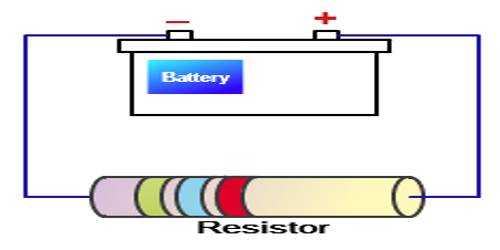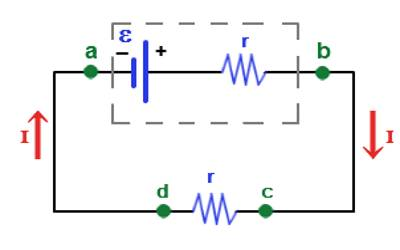Physics

# Induced Electromotive ForceInduced Electromotive Force

We know how induced current is generated in a closed coil in the magnetic field. The very presence of induced current in the coil proves that electromotive force has been induced in it. If there is a small gap i.e., an air gap between the terminals of the coil, instead of the closed coil, there is an electromotive force but there is no flow of current. Now we will see what do we understand by induced electromotive force?

The temporary electromotive force generated due to electromagnetic induction is called induced electromotive force and the temporary current is called the induced current. In a closed circuit, temporary electromotive force and current produced due to the electromagnetic induction are called induced electromotive force and induced current respectively.Induced EMF = – Change in the Magnetic flux/change in time

The equation is: EMF = – ΔΦ /Δt

Where:

EMF: Electromotive force

ΔΦ: Change of the magnetic flux

Δt: change in time